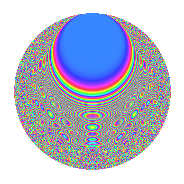Properties

 Label 6007.2.a.cLevel 6007 Weight 2 Character orbit 6007.a Self dual Yes Analytic conductor 47.966 Analytic rank 0 Dimension 261 CM No

Related objects

Newspace parameters

 Level: $$N$$ = $$6007$$ Weight: $$k$$ = $$2$$ Character orbit: $$[\chi]$$ = 6007.a (trivial)

Newform invariants

 Self dual: Yes Analytic conductor: $$47.9661364942$$ Analytic rank: $$0$$ Dimension: $$261$$ Fricke sign: $$-1$$ Sato-Tate group: $\mathrm{SU}(2)$

$q$-expansion

The dimension is sufficiently large that we do not compute an algebraic $$q$$-expansion, but we have computed the trace expansion.

 $$\operatorname{Tr}(f)(q) =$$ $$261q$$ $$\mathstrut +\mathstrut 26q^{2}$$ $$\mathstrut +\mathstrut 25q^{3}$$ $$\mathstrut +\mathstrut 274q^{4}$$ $$\mathstrut +\mathstrut 66q^{5}$$ $$\mathstrut +\mathstrut 25q^{6}$$ $$\mathstrut +\mathstrut 37q^{7}$$ $$\mathstrut +\mathstrut 72q^{8}$$ $$\mathstrut +\mathstrut 310q^{9}$$ $$\mathstrut +\mathstrut O(q^{10})$$ $$\operatorname{Tr}(f)(q) =$$ $$261q$$ $$\mathstrut +\mathstrut 26q^{2}$$ $$\mathstrut +\mathstrut 25q^{3}$$ $$\mathstrut +\mathstrut 274q^{4}$$ $$\mathstrut +\mathstrut 66q^{5}$$ $$\mathstrut +\mathstrut 25q^{6}$$ $$\mathstrut +\mathstrut 37q^{7}$$ $$\mathstrut +\mathstrut 72q^{8}$$ $$\mathstrut +\mathstrut 310q^{9}$$ $$\mathstrut +\mathstrut 35q^{10}$$ $$\mathstrut +\mathstrut 32q^{11}$$ $$\mathstrut +\mathstrut 51q^{12}$$ $$\mathstrut +\mathstrut 60q^{13}$$ $$\mathstrut +\mathstrut 55q^{14}$$ $$\mathstrut +\mathstrut 16q^{15}$$ $$\mathstrut +\mathstrut 288q^{16}$$ $$\mathstrut +\mathstrut 270q^{17}$$ $$\mathstrut +\mathstrut 45q^{18}$$ $$\mathstrut +\mathstrut 34q^{19}$$ $$\mathstrut +\mathstrut 157q^{20}$$ $$\mathstrut +\mathstrut 27q^{21}$$ $$\mathstrut +\mathstrut 38q^{22}$$ $$\mathstrut +\mathstrut 116q^{23}$$ $$\mathstrut +\mathstrut 48q^{24}$$ $$\mathstrut +\mathstrut 335q^{25}$$ $$\mathstrut +\mathstrut 46q^{26}$$ $$\mathstrut +\mathstrut 73q^{27}$$ $$\mathstrut +\mathstrut 70q^{28}$$ $$\mathstrut +\mathstrut 99q^{29}$$ $$\mathstrut +\mathstrut 33q^{30}$$ $$\mathstrut +\mathstrut 33q^{31}$$ $$\mathstrut +\mathstrut 150q^{32}$$ $$\mathstrut +\mathstrut 172q^{33}$$ $$\mathstrut +\mathstrut 24q^{34}$$ $$\mathstrut +\mathstrut 114q^{35}$$ $$\mathstrut +\mathstrut 339q^{36}$$ $$\mathstrut +\mathstrut 36q^{37}$$ $$\mathstrut +\mathstrut 112q^{38}$$ $$\mathstrut +\mathstrut 30q^{39}$$ $$\mathstrut +\mathstrut 106q^{40}$$ $$\mathstrut +\mathstrut 209q^{41}$$ $$\mathstrut +\mathstrut 64q^{42}$$ $$\mathstrut +\mathstrut 64q^{43}$$ $$\mathstrut +\mathstrut 65q^{44}$$ $$\mathstrut +\mathstrut 153q^{45}$$ $$\mathstrut +\mathstrut 135q^{47}$$ $$\mathstrut +\mathstrut 87q^{48}$$ $$\mathstrut +\mathstrut 332q^{49}$$ $$\mathstrut +\mathstrut 82q^{50}$$ $$\mathstrut +\mathstrut 52q^{51}$$ $$\mathstrut +\mathstrut 102q^{52}$$ $$\mathstrut +\mathstrut 163q^{53}$$ $$\mathstrut +\mathstrut 52q^{54}$$ $$\mathstrut +\mathstrut 56q^{55}$$ $$\mathstrut +\mathstrut 134q^{56}$$ $$\mathstrut +\mathstrut 181q^{57}$$ $$\mathstrut +\mathstrut q^{58}$$ $$\mathstrut +\mathstrut 89q^{59}$$ $$\mathstrut -\mathstrut 43q^{60}$$ $$\mathstrut +\mathstrut 112q^{61}$$ $$\mathstrut +\mathstrut 228q^{62}$$ $$\mathstrut +\mathstrut 130q^{63}$$ $$\mathstrut +\mathstrut 268q^{64}$$ $$\mathstrut +\mathstrut 248q^{65}$$ $$\mathstrut +\mathstrut 5q^{66}$$ $$\mathstrut +\mathstrut 42q^{67}$$ $$\mathstrut +\mathstrut 453q^{68}$$ $$\mathstrut +\mathstrut 51q^{69}$$ $$\mathstrut -\mathstrut 22q^{70}$$ $$\mathstrut +\mathstrut 98q^{71}$$ $$\mathstrut +\mathstrut 113q^{72}$$ $$\mathstrut +\mathstrut 206q^{73}$$ $$\mathstrut +\mathstrut 81q^{74}$$ $$\mathstrut +\mathstrut 29q^{75}$$ $$\mathstrut +\mathstrut 62q^{76}$$ $$\mathstrut +\mathstrut 185q^{77}$$ $$\mathstrut -\mathstrut 25q^{78}$$ $$\mathstrut +\mathstrut 29q^{79}$$ $$\mathstrut +\mathstrut 258q^{80}$$ $$\mathstrut +\mathstrut 393q^{81}$$ $$\mathstrut +\mathstrut 79q^{82}$$ $$\mathstrut +\mathstrut 265q^{83}$$ $$\mathstrut -\mathstrut 25q^{84}$$ $$\mathstrut +\mathstrut 84q^{85}$$ $$\mathstrut +\mathstrut 36q^{86}$$ $$\mathstrut +\mathstrut 131q^{87}$$ $$\mathstrut +\mathstrut 24q^{88}$$ $$\mathstrut +\mathstrut 195q^{89}$$ $$\mathstrut +\mathstrut 89q^{90}$$ $$\mathstrut -\mathstrut 18q^{91}$$ $$\mathstrut +\mathstrut 261q^{92}$$ $$\mathstrut +\mathstrut 52q^{93}$$ $$\mathstrut +\mathstrut 3q^{94}$$ $$\mathstrut +\mathstrut 104q^{95}$$ $$\mathstrut +\mathstrut 92q^{96}$$ $$\mathstrut +\mathstrut 213q^{97}$$ $$\mathstrut +\mathstrut 156q^{98}$$ $$\mathstrut +\mathstrut 47q^{99}$$ $$\mathstrut +\mathstrut O(q^{100})$$

Embeddings

For each embedding $$\iota_m$$ of the coefficient field, the values $$\iota_m(a_n)$$ are shown below.

For more information on an embedded modular form you can click on its label.

Label $$a_{2}$$ $$a_{3}$$ $$a_{4}$$ $$a_{5}$$ $$a_{6}$$ $$a_{7}$$ $$a_{8}$$ $$a_{9}$$ $$a_{10}$$
1.1 −2.82040 1.97504 5.95465 −2.55663 −5.57039 −3.03915 −11.1537 0.900772 7.21072
1.2 −2.81023 −2.20286 5.89742 4.33627 6.19056 0.601928 −10.9527 1.85260 −12.1859
1.3 −2.72871 0.0576599 5.44587 −1.32868 −0.157337 0.160488 −9.40280 −2.99668 3.62560
1.4 −2.68856 2.47306 5.22837 0.0319975 −6.64899 3.92320 −8.67966 3.11605 −0.0860273
1.5 −2.66623 −2.56917 5.10878 0.348811 6.85001 3.60714 −8.28872 3.60066 −0.930012
1.6 −2.65529 2.71142 5.05057 3.32212 −7.19960 −2.23518 −8.10014 4.35179 −8.82118
1.7 −2.63601 0.145552 4.94856 0.530894 −0.383678 0.464377 −7.77243 −2.97881 −1.39944
1.8 −2.63092 0.625555 4.92176 3.53160 −1.64579 2.86224 −7.68693 −2.60868 −9.29136
1.9 −2.57859 −1.33205 4.64914 0.797435 3.43482 −0.447865 −6.83106 −1.22564 −2.05626
1.10 −2.56111 −3.17696 4.55927 0.542831 8.13653 −2.10285 −6.55456 7.09306 −1.39025
1.11 −2.55274 −1.51769 4.51649 −0.0618695 3.87427 −0.267248 −6.42394 −0.696618 0.157937
1.12 −2.53967 2.55882 4.44992 2.30710 −6.49855 −1.02038 −6.22199 3.54756 −5.85928
1.13 −2.53843 −2.17354 4.44361 −2.13295 5.51736 −4.01617 −6.20293 1.72426 5.41433
1.14 −2.52735 0.935367 4.38748 −0.964544 −2.36400 −4.26765 −6.03399 −2.12509 2.43774
1.15 −2.52396 2.90244 4.37039 −2.11388 −7.32566 −3.46277 −5.98279 5.42417 5.33537
1.16 −2.49977 0.0230289 4.24887 3.35515 −0.0575671 0.906816 −5.62166 −2.99947 −8.38713
1.17 −2.47861 −3.39135 4.14353 2.04119 8.40584 3.41777 −5.31299 8.50123 −5.05931
1.18 −2.47715 2.24713 4.13629 −0.500546 −5.56650 2.06873 −5.29193 2.04961 1.23993
1.19 −2.47082 3.41011 4.10496 1.44488 −8.42576 2.75509 −5.20098 8.62882 −3.57005
1.20 −2.46886 −1.31806 4.09526 2.84222 3.25411 −1.17521 −5.17289 −1.26271 −7.01703
See next 80 embeddings (of 261 total)
 $$n$$: e.g. 2-40 or 990-1000 Embeddings: e.g. 1-3 or 1.261 Significant digits: Format: Complex embeddings Normalized embeddings Satake parameters Satake angles

Inner twists

This newform does not have CM; other inner twists have not been computed.

Atkin-Lehner signs

$$p$$ Sign
$$6007$$ $$-1$$

Hecke kernels

This newform can be constructed as the kernel of the linear operator $$T_{2}^{261} - \cdots$$ acting on $$S_{2}^{\mathrm{new}}(\Gamma_0(6007))$$.